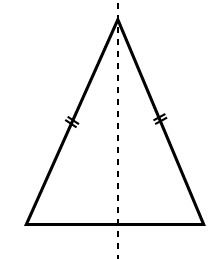# Selina Solutions Concise Maths Class 7 Chapter 17: Symmetry (Including Reflection and Rotation)

Selina Solutions Concise Maths Class 7 Chapter 17 Symmetry (Including Reflection and Rotation) explains symmetric figures and the line of symmetry required for reflection and rotation. A geometric figure is symmetric if the line of symmetry divides it into two identical parts. For further ideas about these concepts, Selina Solutions Concise Maths Class 7 Chapter 17 Symmetry (Including Reflection and Rotation), PDF can be downloaded from the links which are available below.

Chapter 17 discusses the lines of symmetry of given geometrical figures, its reflection and rotation. The solutions PDF can be referred by the students either online or offline mode, based on their necessities.

## Selina Solutions Concise Maths Class 7 Chapter 17: Symmetry (Including Reflection and Rotation) Download PDF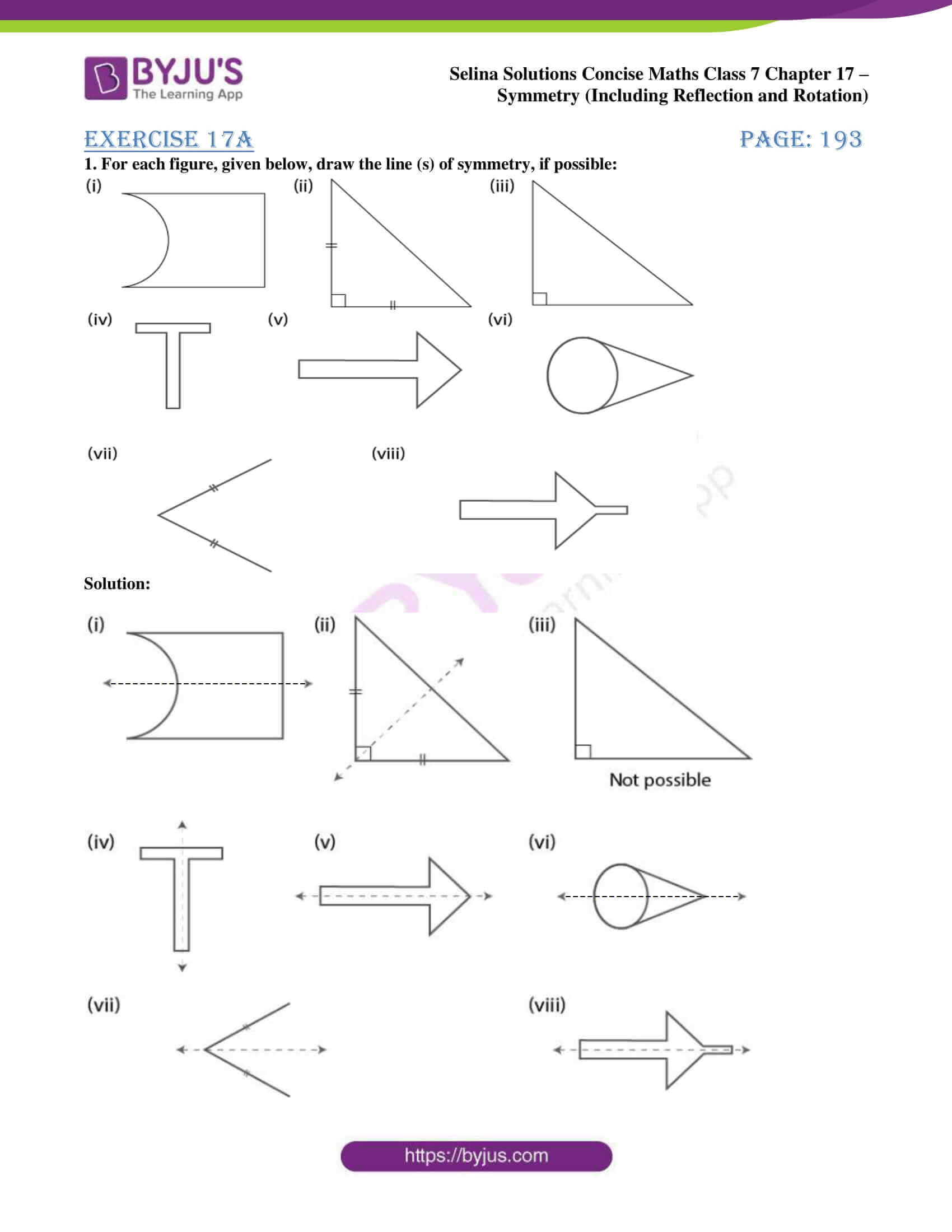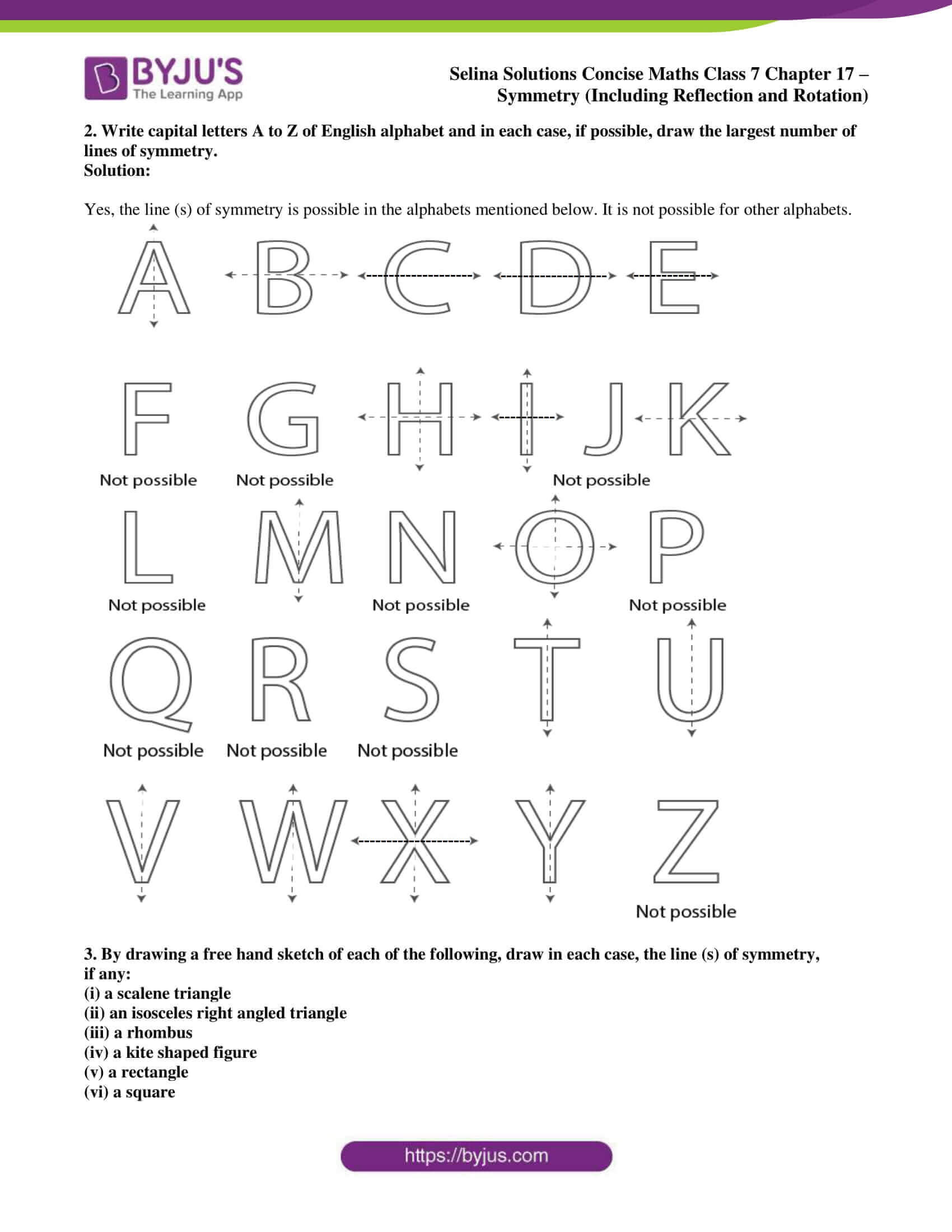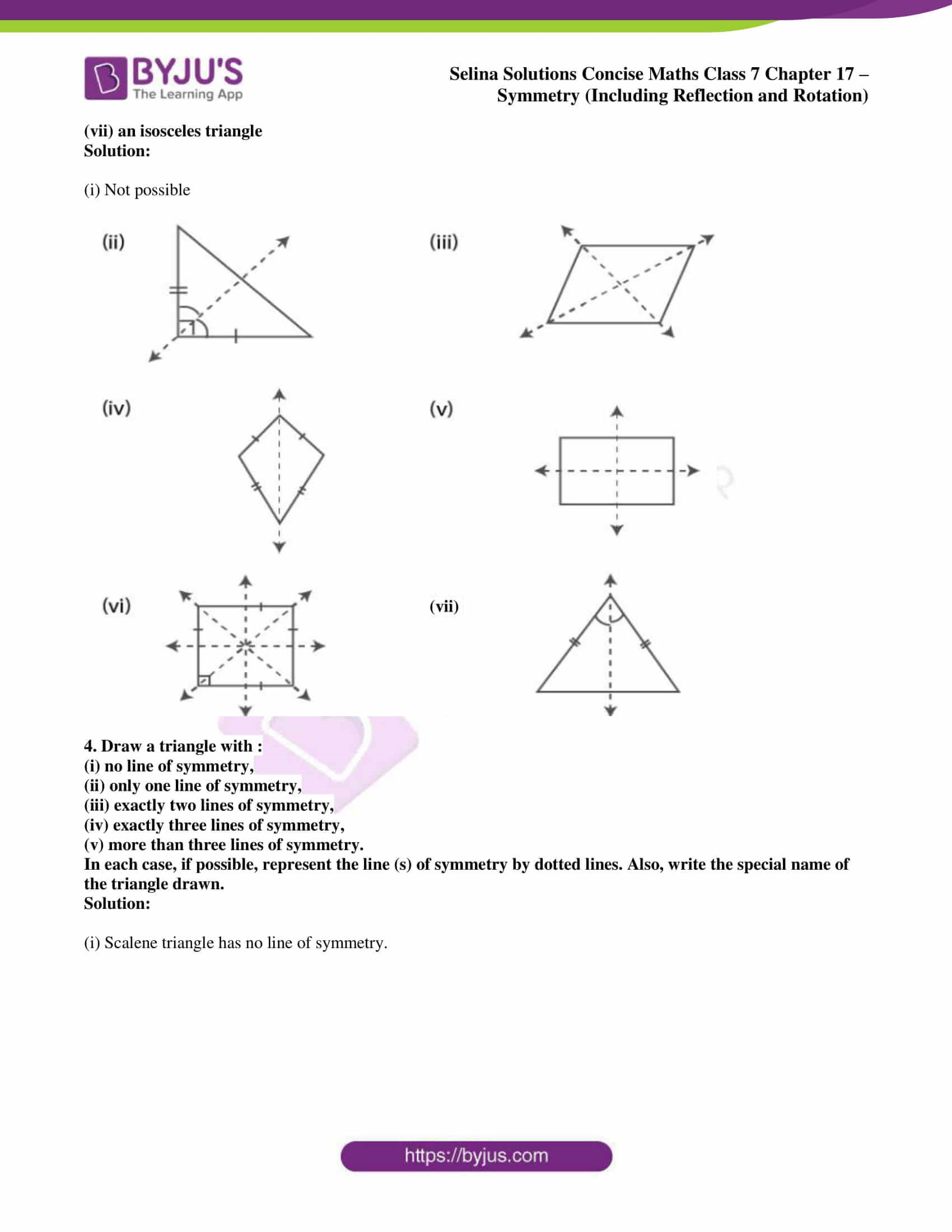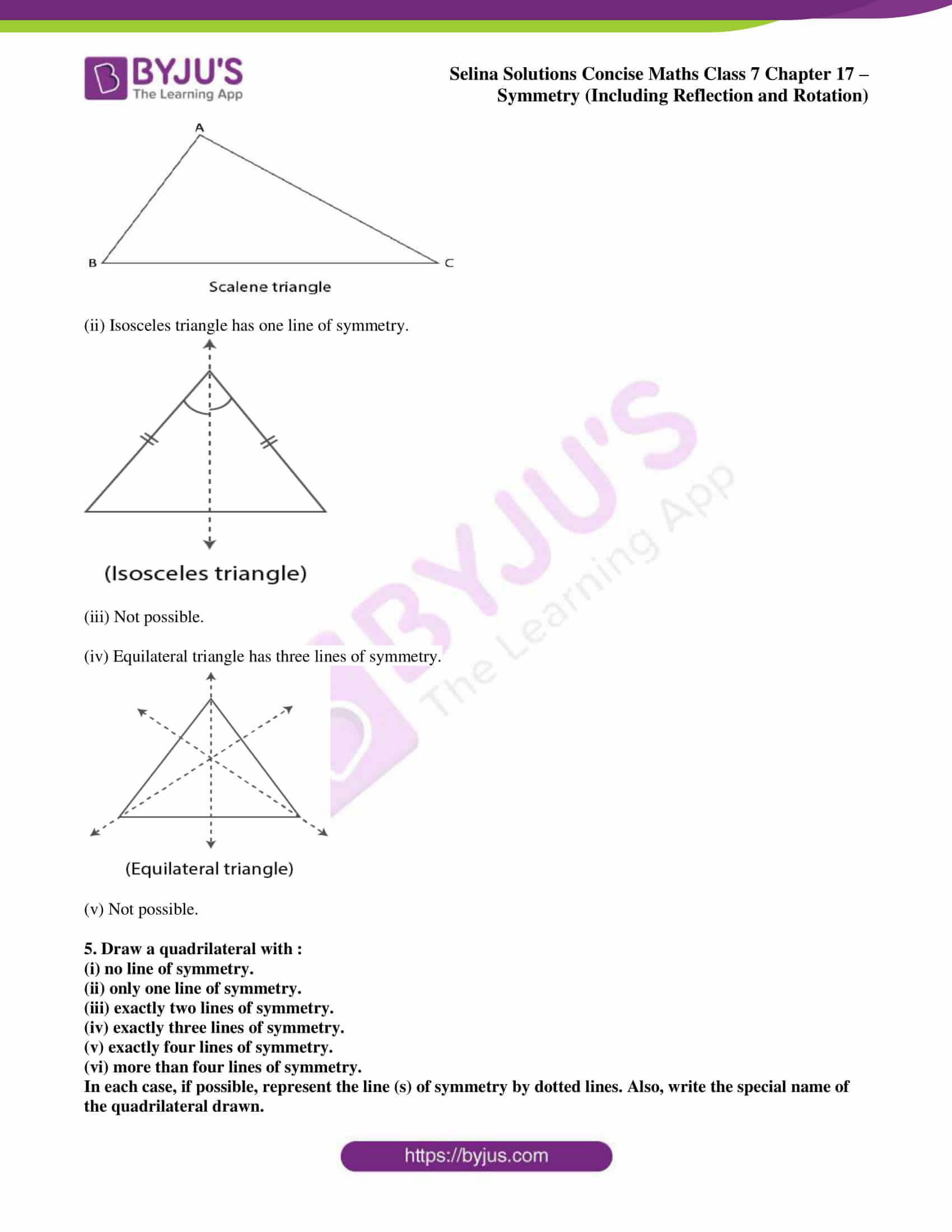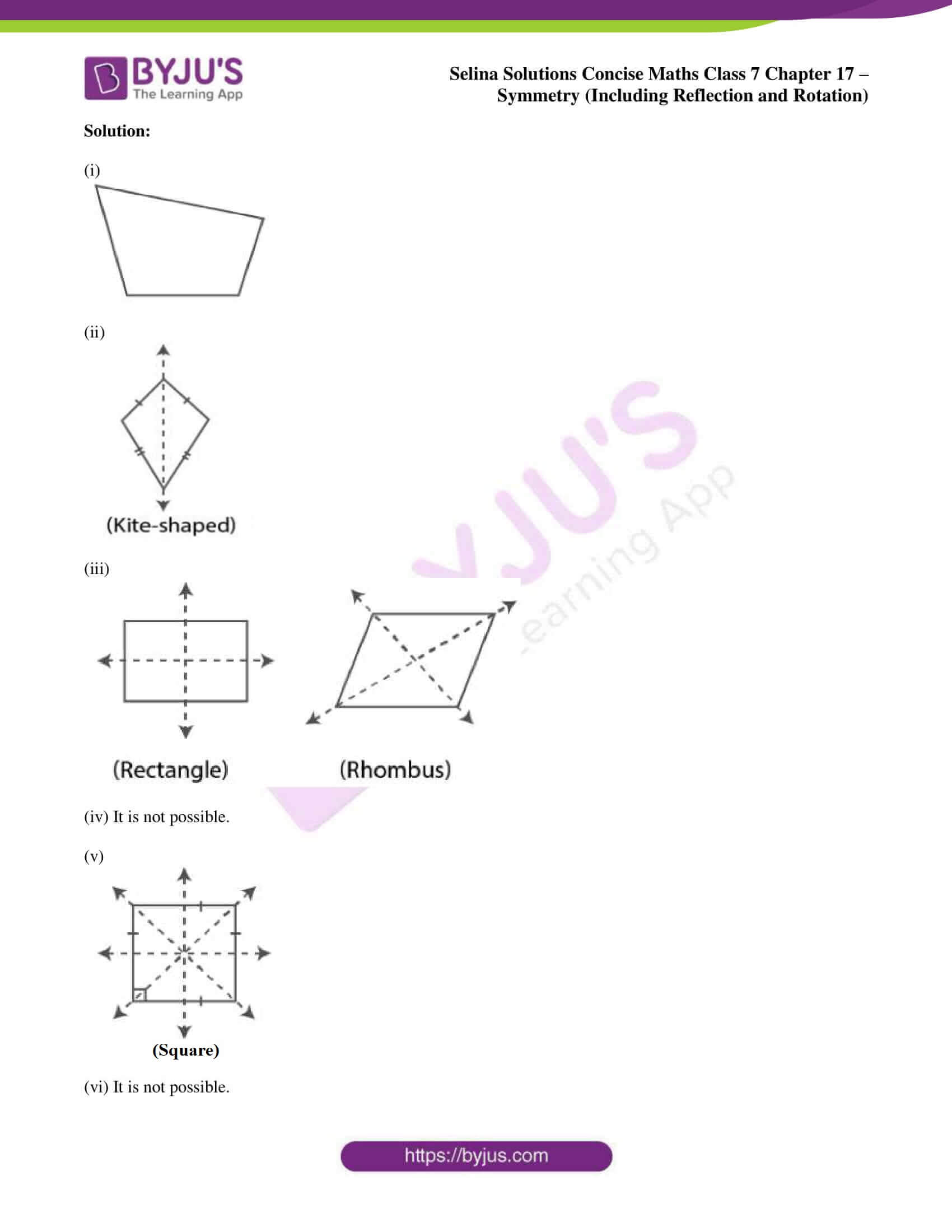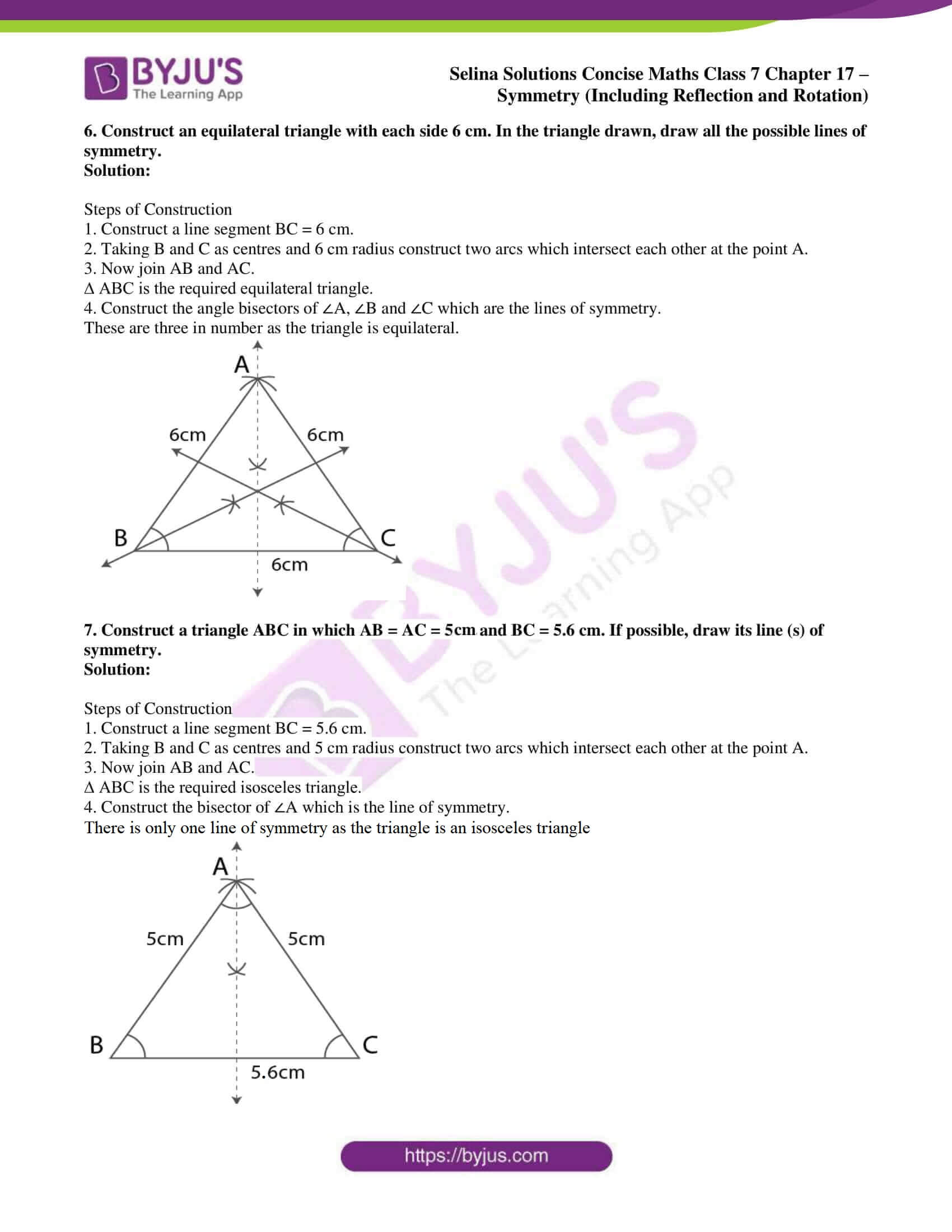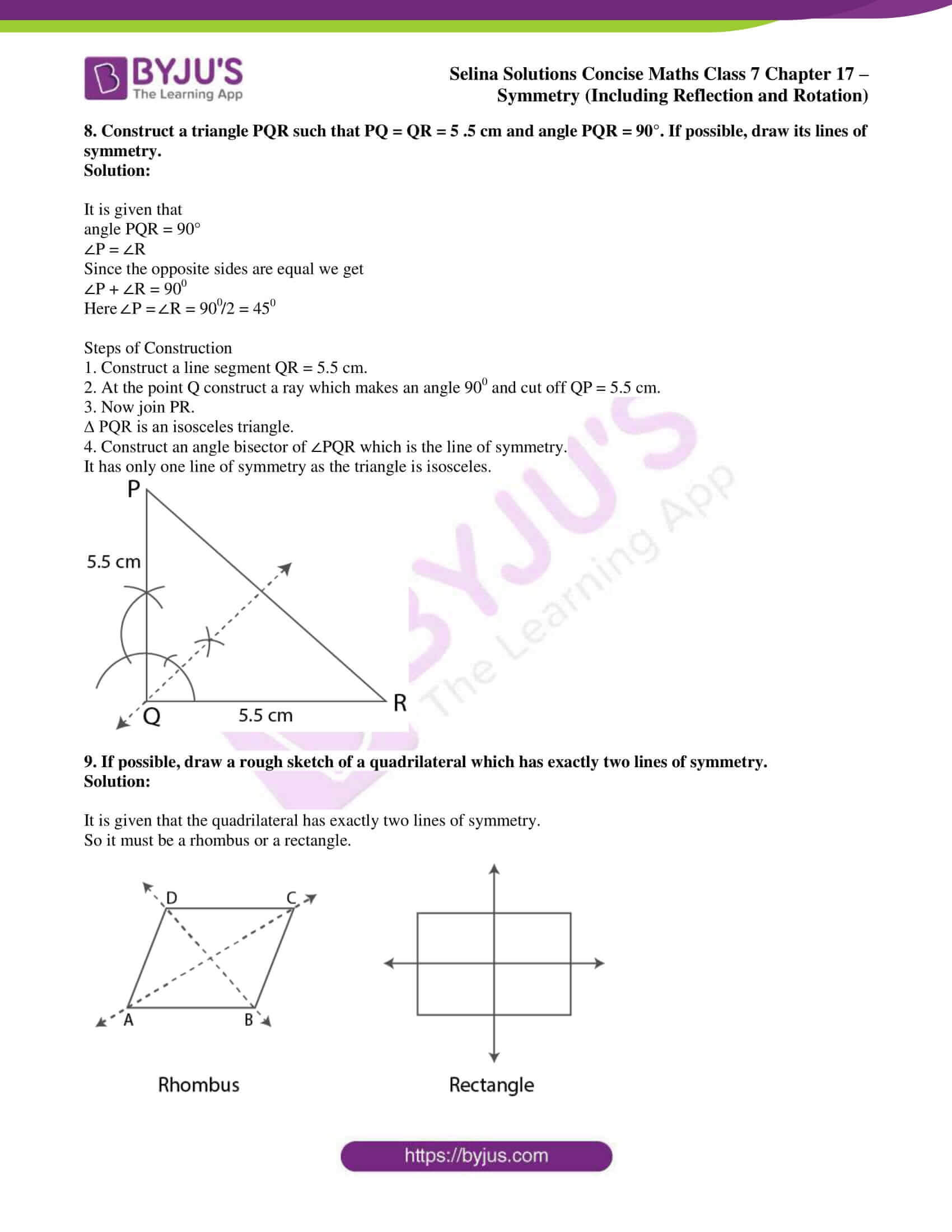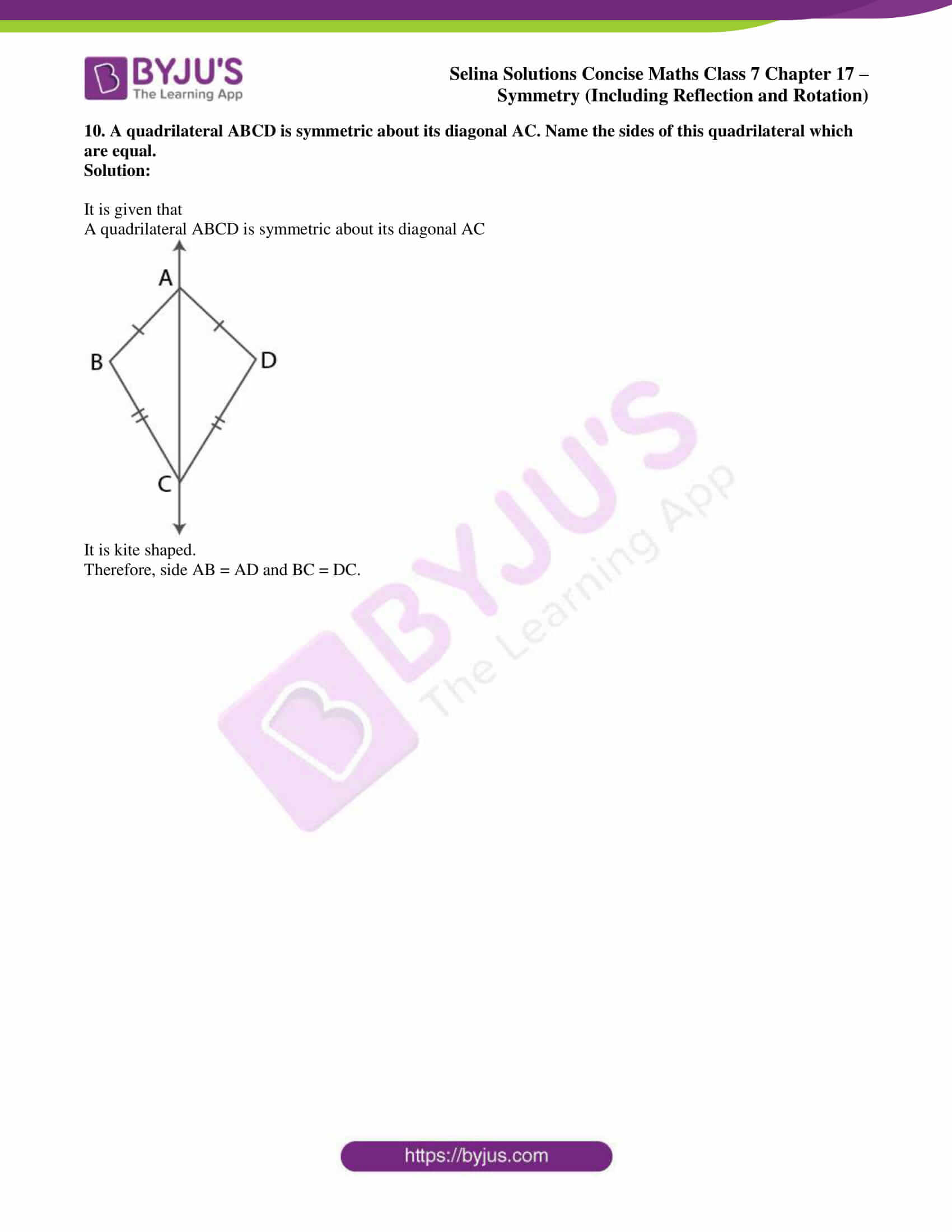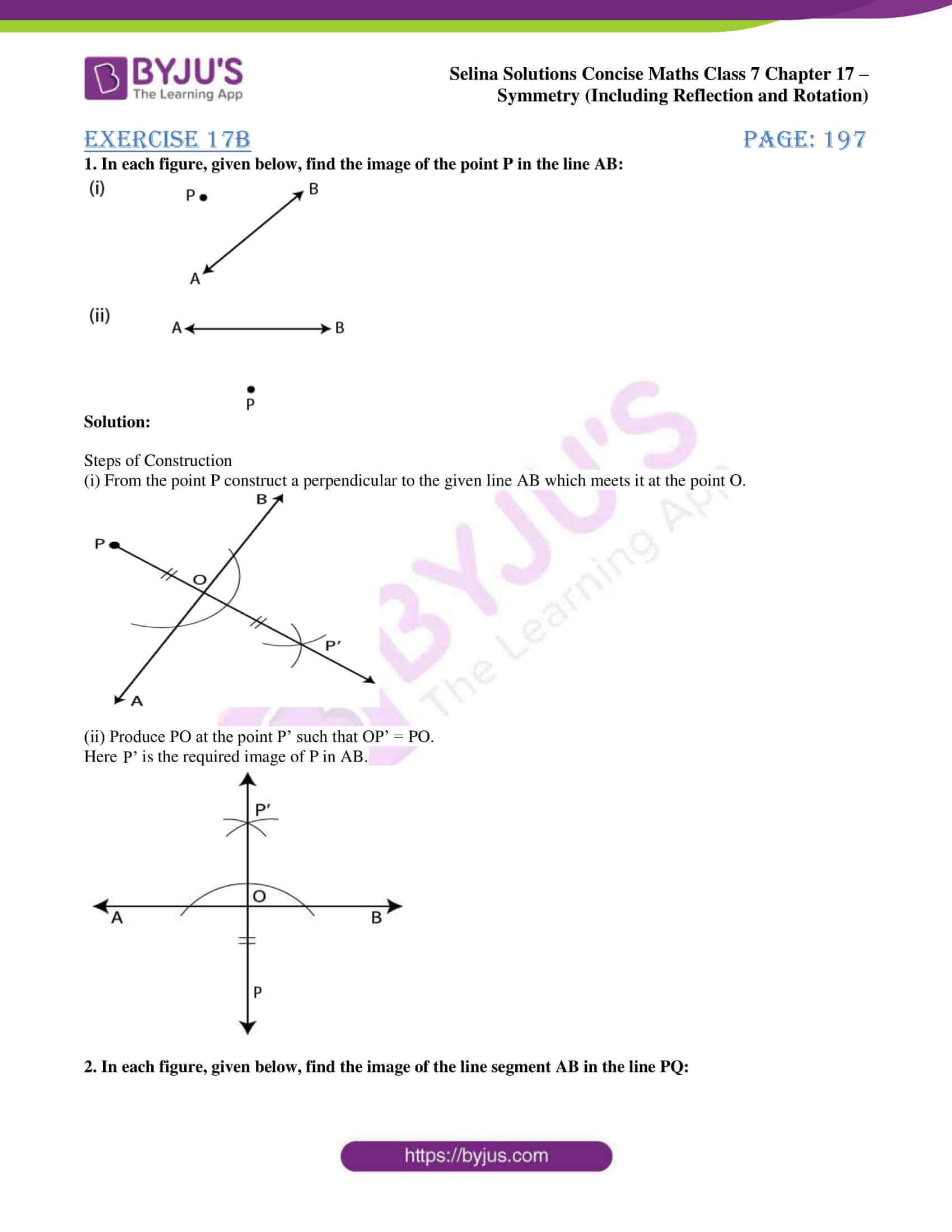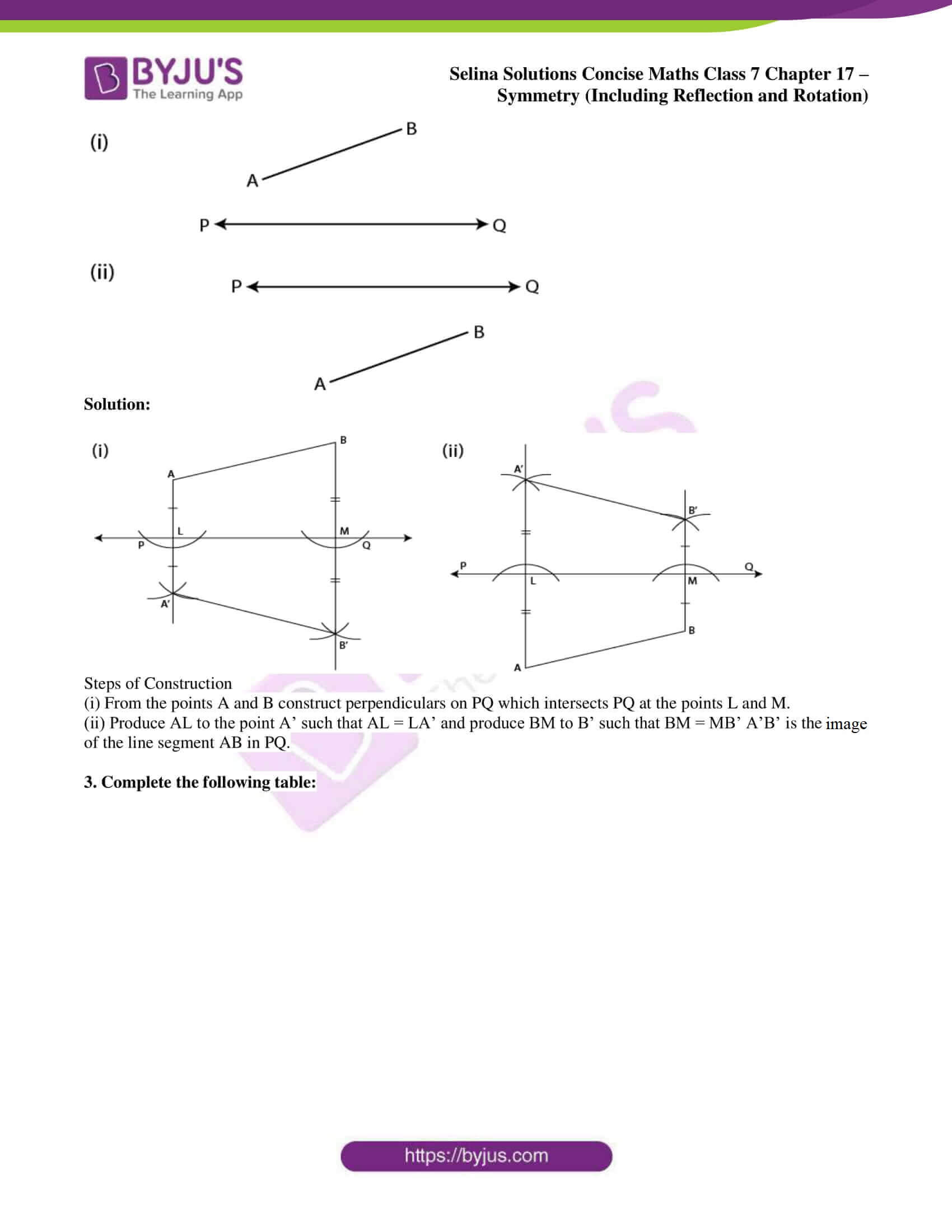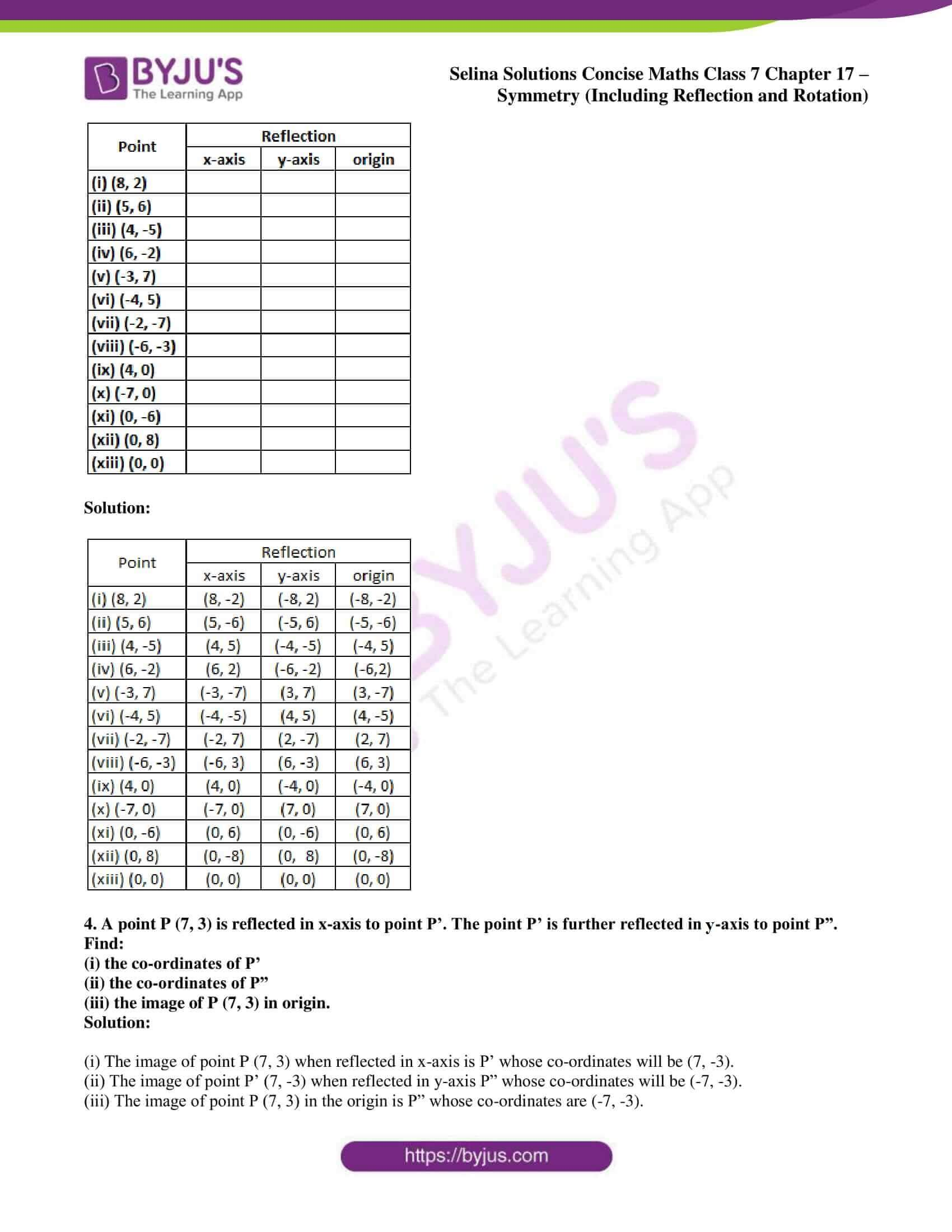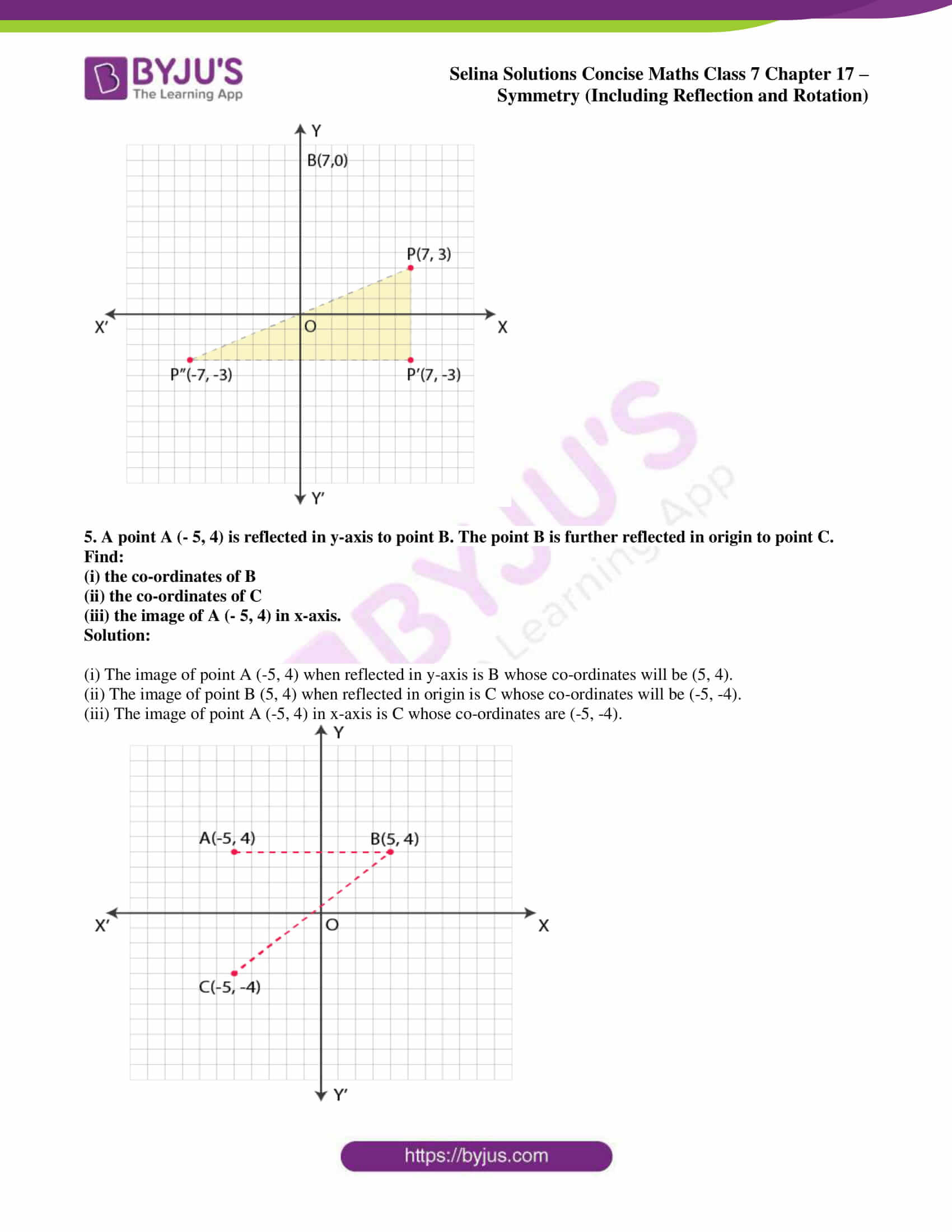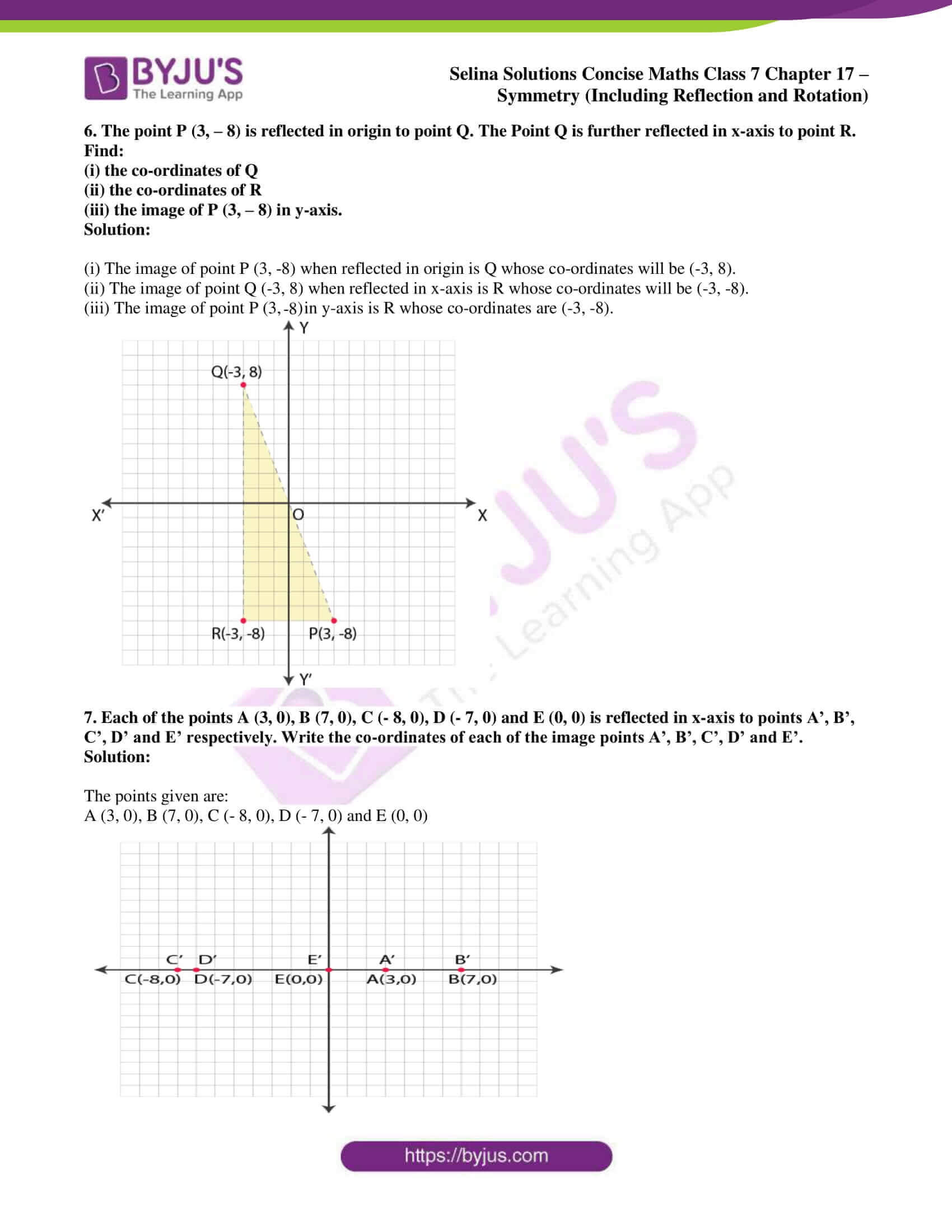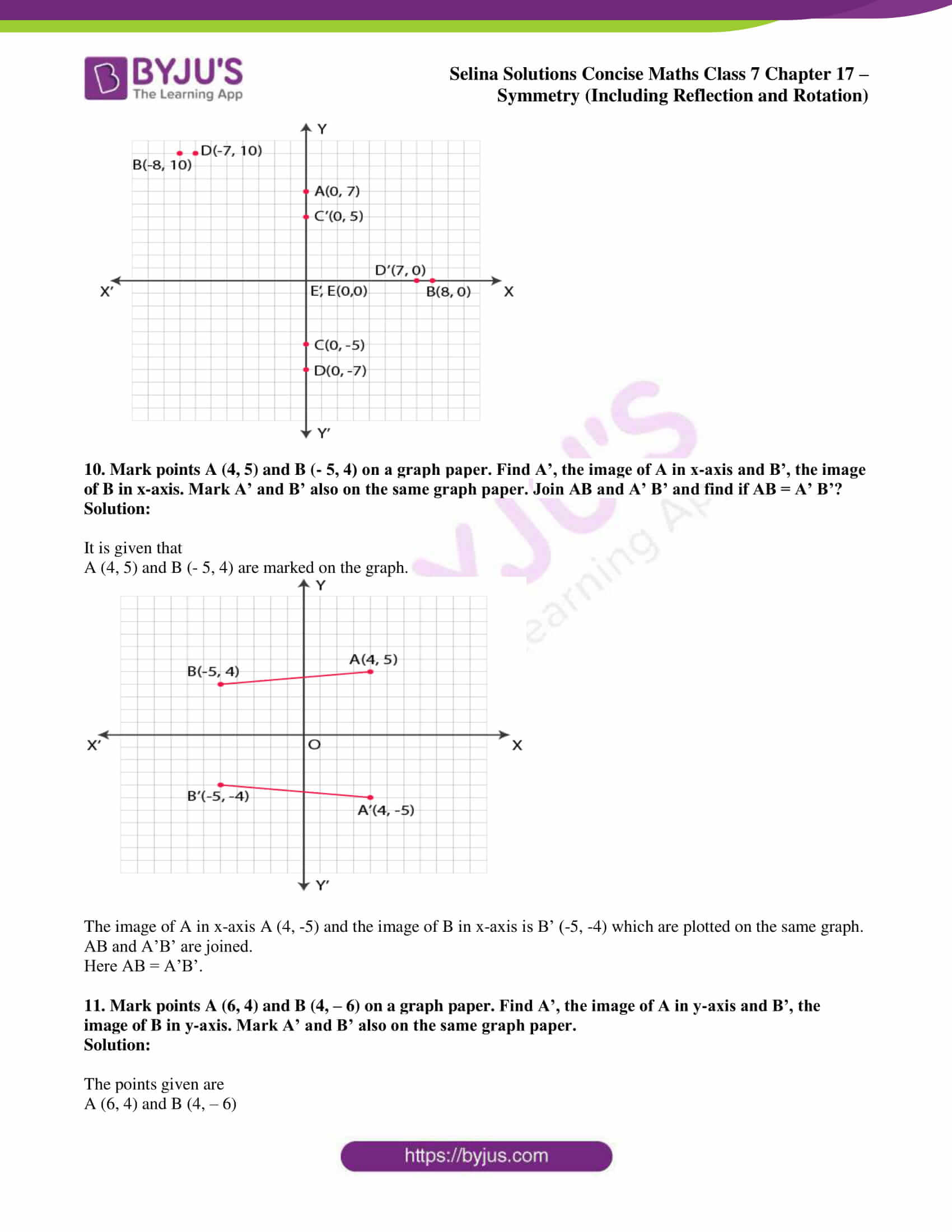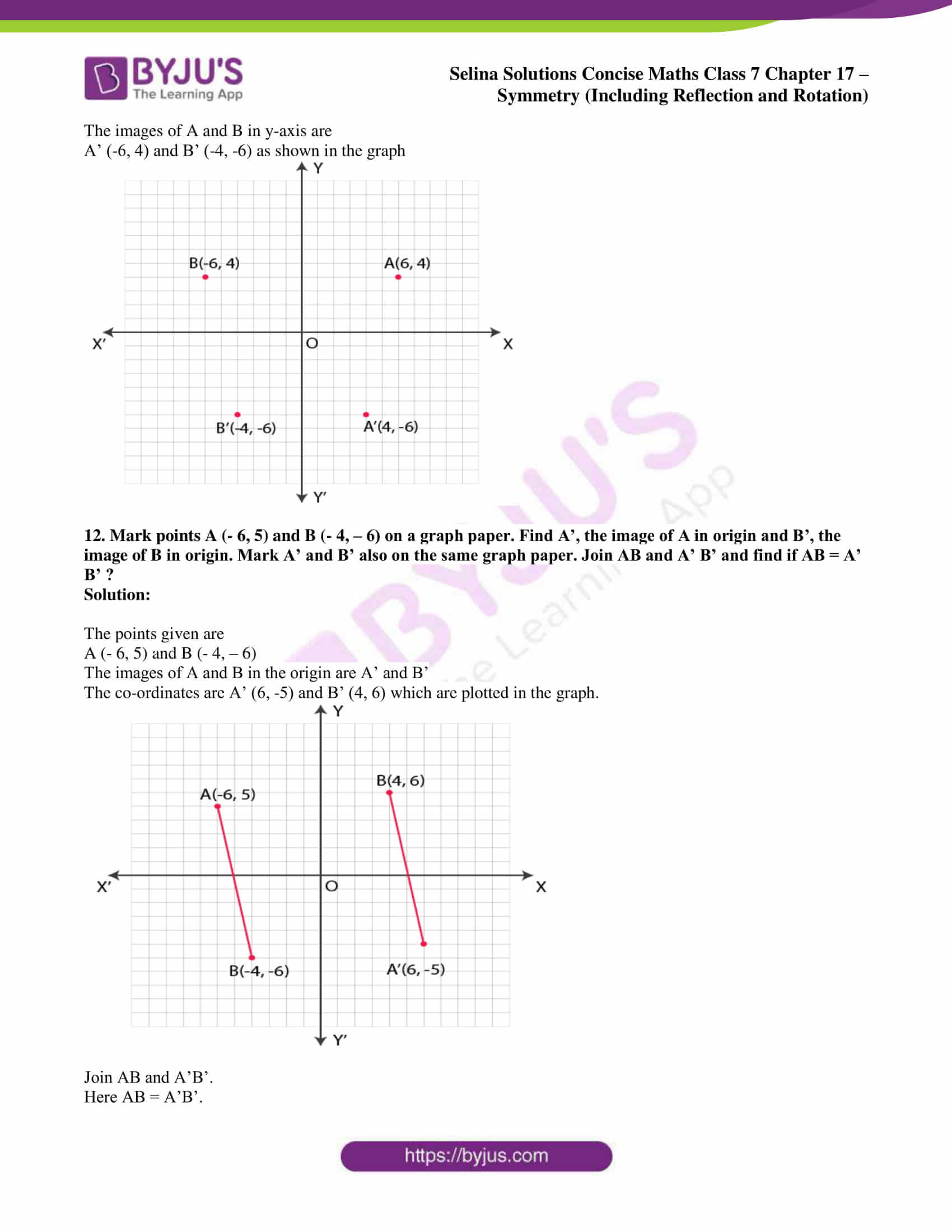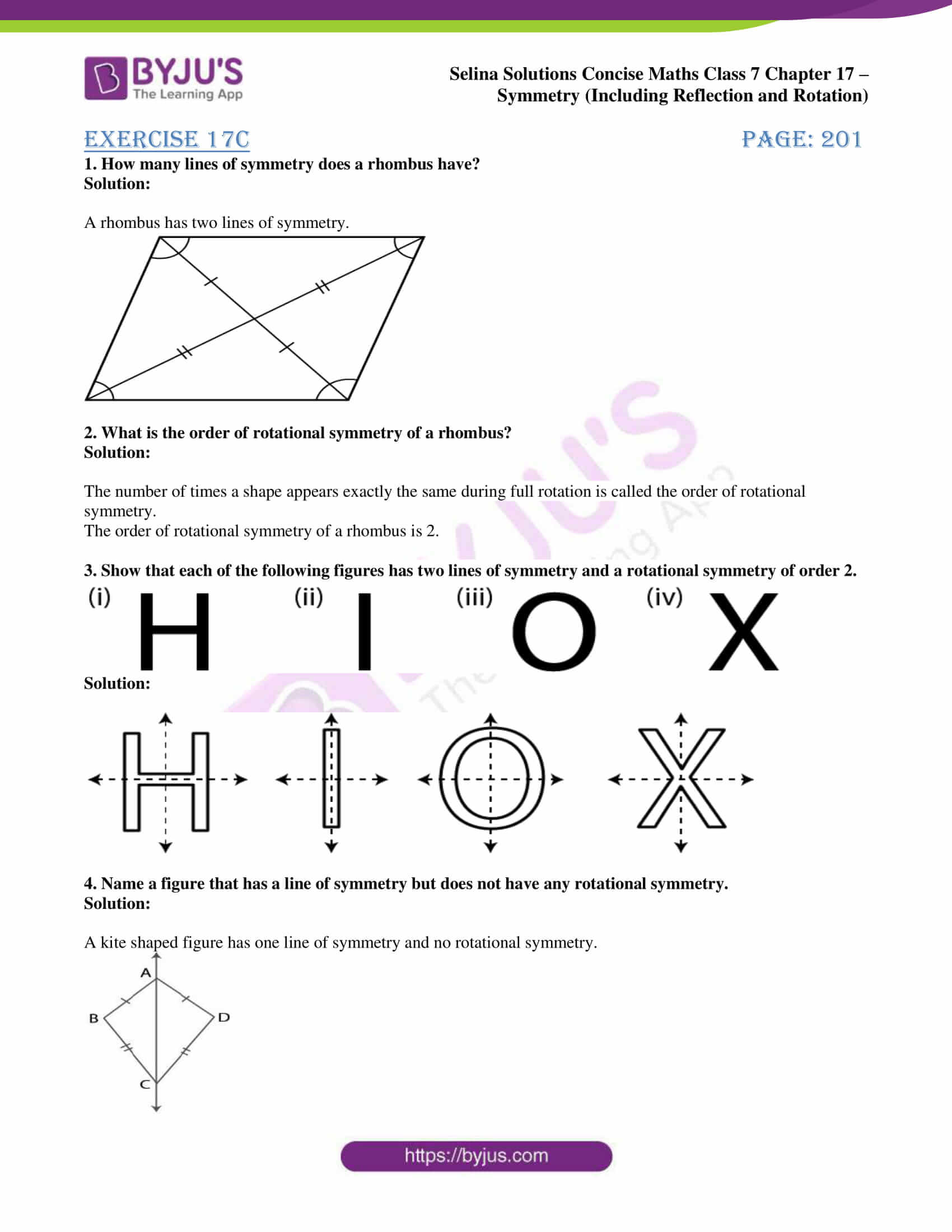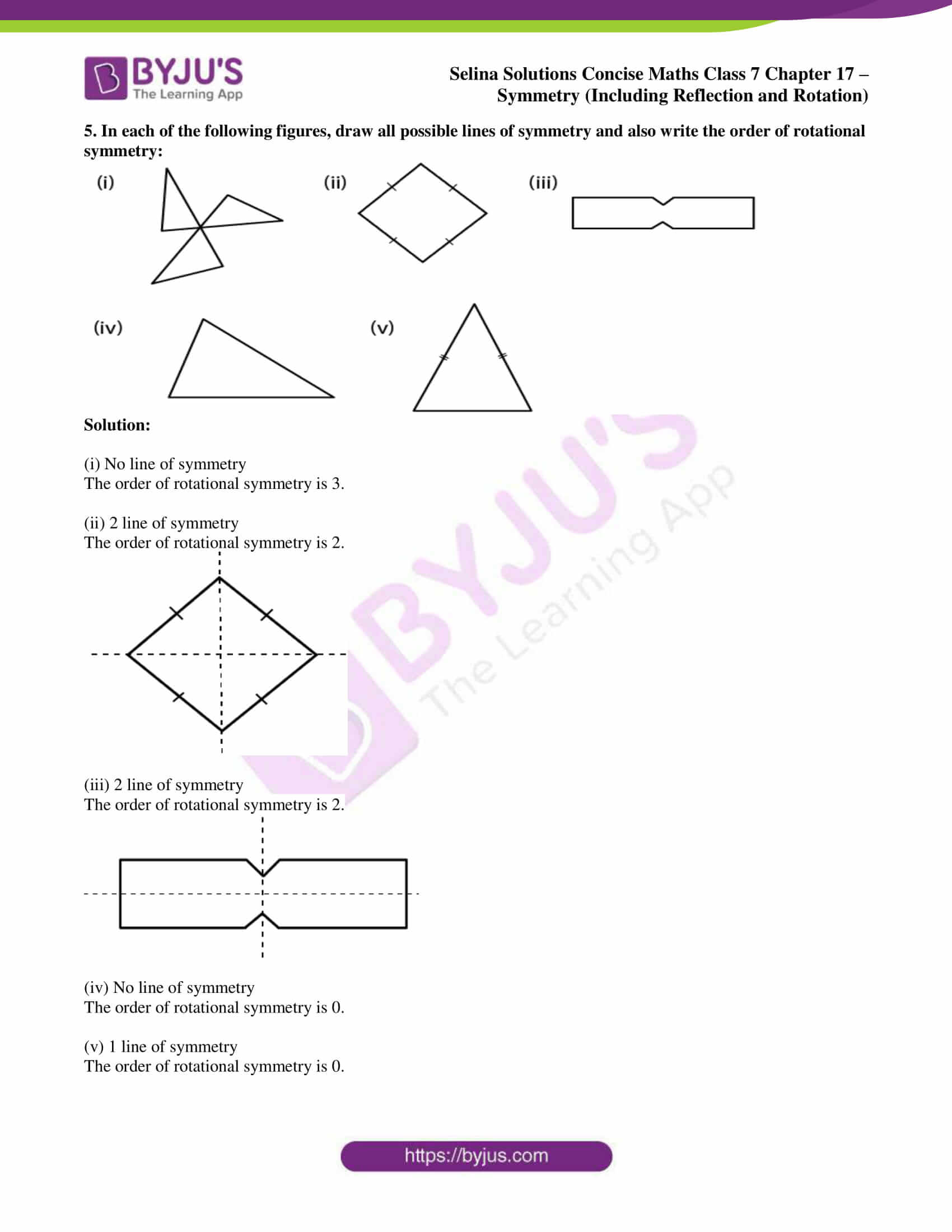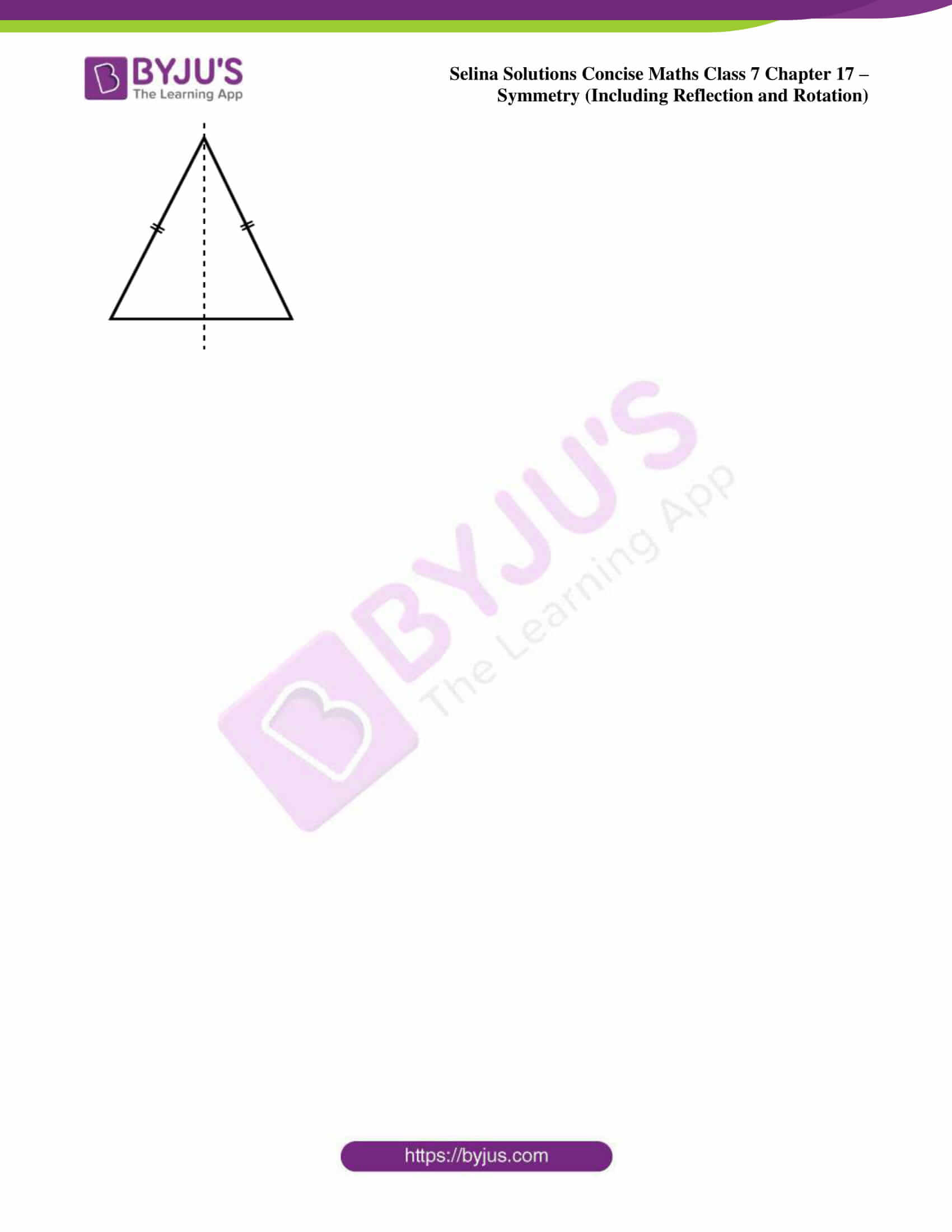### Exercises of Selina Solutions Concise Maths Class 7 Chapter 17 – Symmetry (Including Reflection and Rotation)

Exercise 17A Solutions

Exercise 17B Solutions

Exercise 17C Solutions

## Access Selina Solutions Concise Maths Class 7 Chapter 17: Symmetry (Including Reflection and Rotation)

#### Exercise 17A page: 193

1. For each figure, given below, draw the line (s) of symmetry, if possible: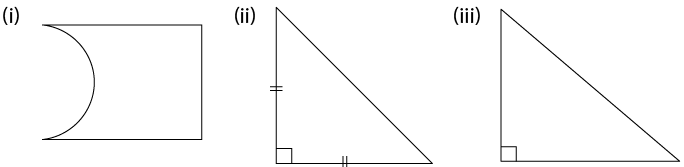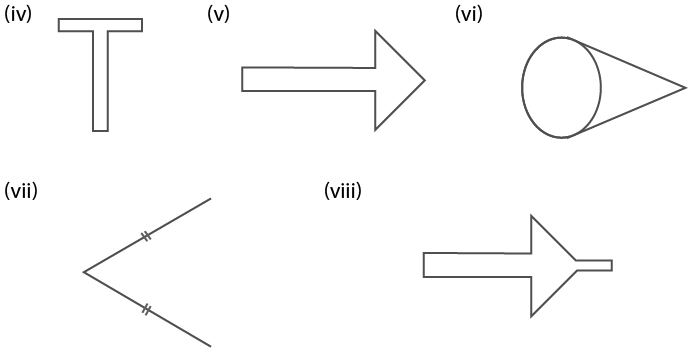Solution: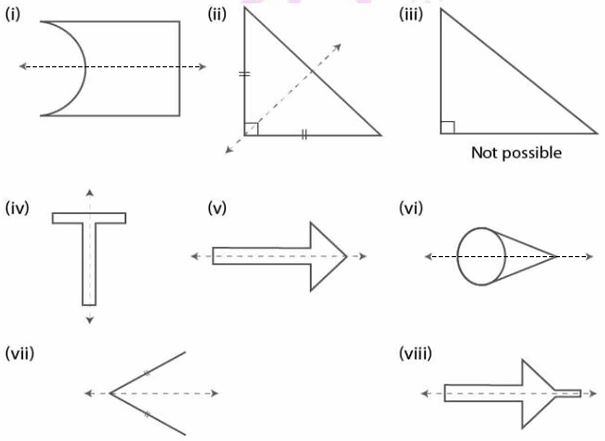2. Write capital letters A to Z of English alphabet and in each case, if possible, draw the largest number of lines of symmetry.

Solution:

Yes, the line (s) of symmetry is possible in the alphabets mentioned below. It is not possible for other alphabets.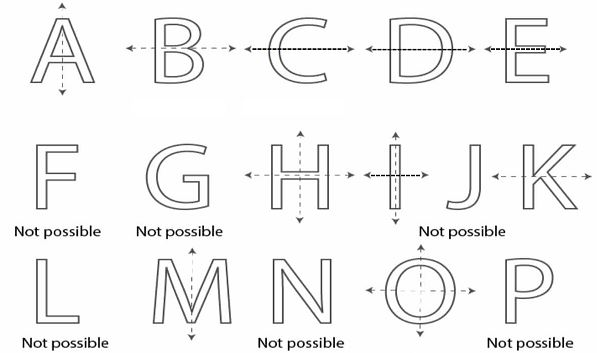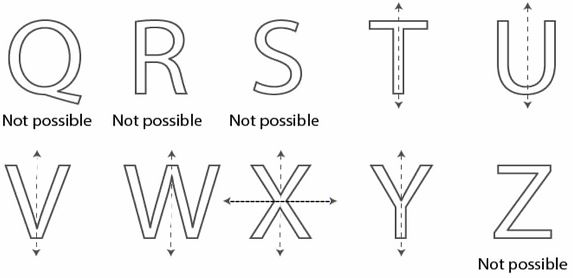3. By drawing a free hand sketch of each of the following, draw in each case, the line (s) of symmetry,

if any:

(i) a scalene triangle

(ii) an isosceles right angled triangle

(iii) a rhombus

(iv) a kite shaped figure

(v) a rectangle

(vi) a square

(vii) an isosceles triangle

Solution:

(i) Not possible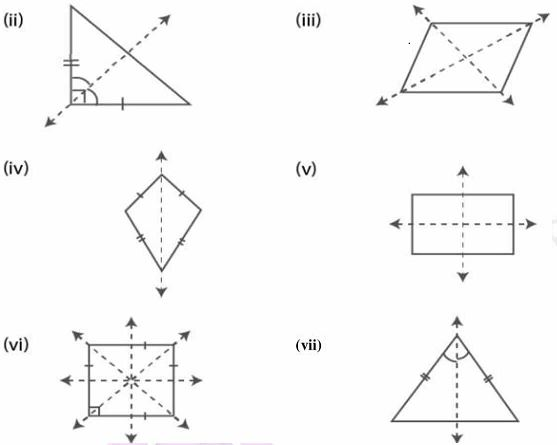4. Draw a triangle with :

(i) no line of symmetry,

(ii) only one line of symmetry,

(iii) exactly two lines of symmetry,

(iv) exactly three lines of symmetry,

(v) more than three lines of symmetry.

In each case, if possible, represent the line (s) of symmetry by dotted lines. Also, write the special name of the triangle drawn.

Solution:

(i) Scalene triangle has no line of symmetry.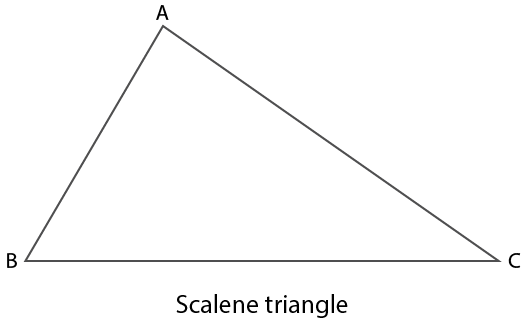(ii) Isosceles triangle has one line of symmetry.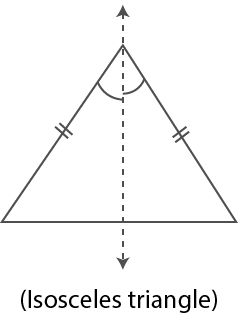(iii) Not possible.

(iv) Equilateral triangle has three lines of symmetry.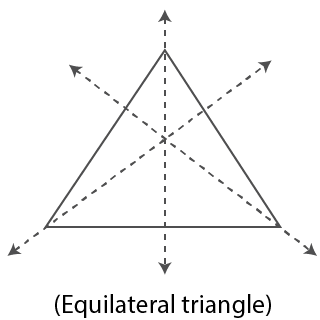(v) Not possible.

5. Draw a quadrilateral with :

(i) no line of symmetry.

(ii) only one line of symmetry.

(iii) exactly two lines of symmetry.

(iv) exactly three lines of symmetry.

(v) exactly four lines of symmetry.

(vi) more than four lines of symmetry.

In each case, if possible, represent the line (s) of symmetry by dotted lines. Also, write the special name of the quadrilateral drawn.

Solution:

(i)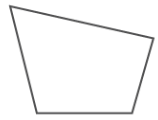(ii)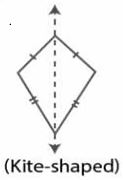(iii)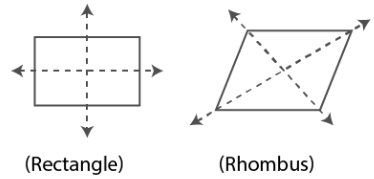(iv) It is not possible.

(v)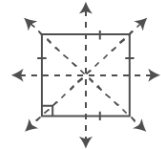(Square)

(vi) It is not possible.

6. Construct an equilateral triangle with each side 6 cm. In the triangle drawn, draw all the possible lines of symmetry.

Solution:

Steps of Construction

1. Construct a line segment BC = 6 cm.

2. Taking B and C as centres and 6 cm radius construct two arcs which intersect each other at the point A.

3. Now join AB and AC.

∆ ABC is the required equilateral triangle.

4. Construct the angle bisectors of ∠A, ∠B and ∠C which are the lines of symmetry.

These are three in number as the triangle is equilateral.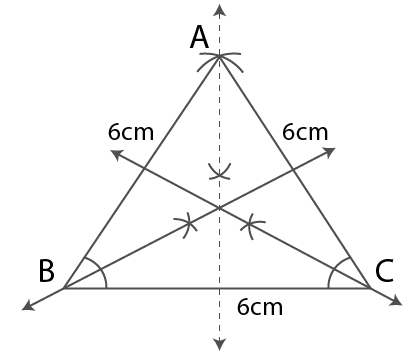7. Construct a triangle ABC in which AB = AC = 5cm and BC = 5.6 cm. If possible, draw its line (s) of symmetry.

Solution:

Steps of Construction

1. Construct a line segment BC = 5.6 cm.

2. Taking B and C as centres and 5 cm radius construct two arcs which intersect each other at the point A.

3. Now join AB and AC.

∆ ABC is the required isosceles triangle.

4. Construct the bisector of ∠A which is the line of symmetry.

There is only one line of symmetry as the triangle is an isosceles triangle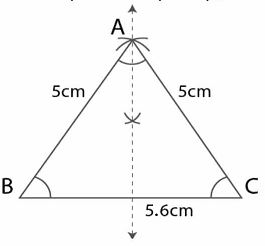8. Construct a triangle PQR such that PQ = QR = 5 .5 cm and angle PQR = 90°. If possible, draw its lines of symmetry.

Solution:

It is given that

angle PQR = 90°

∠P = ∠R

Since the opposite sides are equal we get

∠P + ∠R = 900

Here ∠P = ∠R = 90/2 = 450

Steps of Construction

1. Construct a line segment QR = 5.5 cm.

2. At the point Q construct a ray which makes an angle 900 and cut off QP = 5.5 cm.

3. Now join PR.

∆ PQR is an isosceles triangle.

4. Construct an angle bisector of ∠PQR which is the line of symmetry.

It has only one line of symmetry as the triangle is isosceles.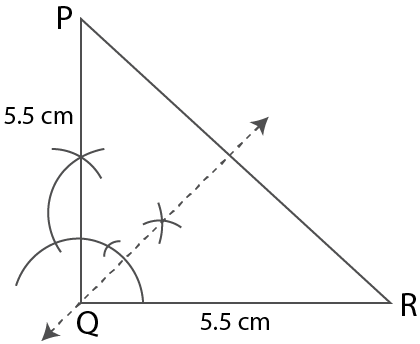9. If possible, draw a rough sketch of a quadrilateral which has exactly two lines of symmetry.

Solution:

It is given that the quadrilateral has exactly two lines of symmetry.

So it must be a rhombus or a rectangle.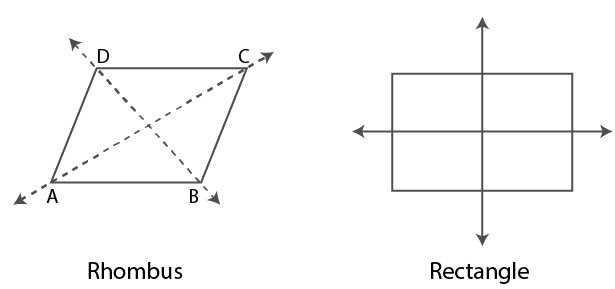10. A quadrilateral ABCD is symmetric about its diagonal AC. Name the sides of this quadrilateral which are equal.

Solution:

It is given that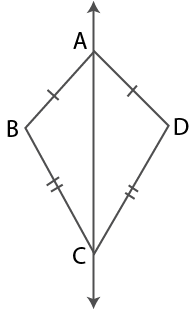It is kite shaped.

Therefore, side AB = AD and BC = DC.

#### Exercise 17B PAGE: 197

1. In each figure, given below, find the image of the point P in the line AB: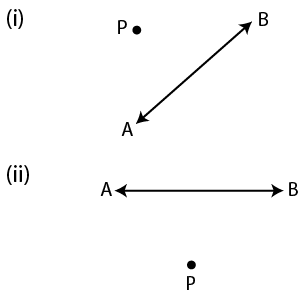Solution:

Steps of Construction

(i) From the point P construct a perpendicular to the given line AB which meets it at the point O.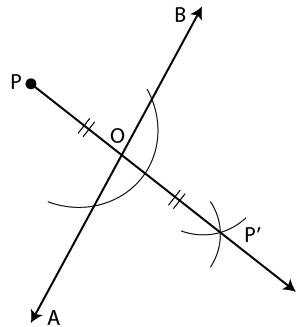(ii) Produce PO at the point P’ such that OP’ = PO.

Here P’ is the required image of P in AB.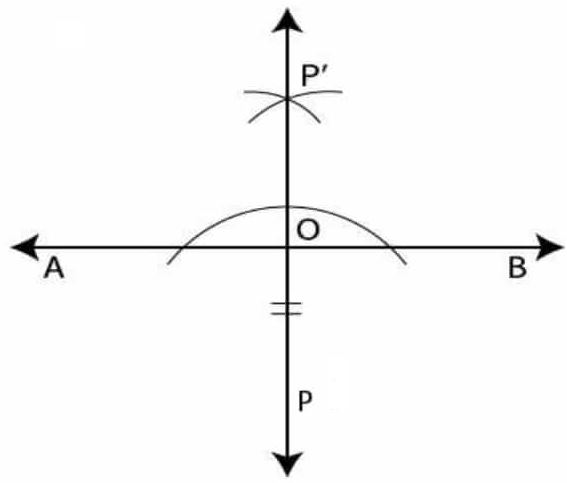2. In each figure, given below, find the image of the line segment AB in the line PQ: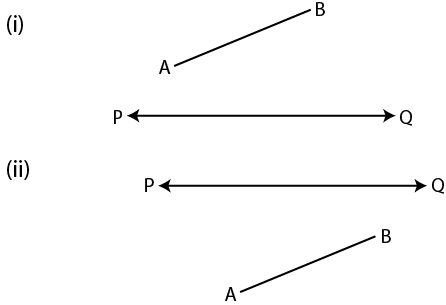Solution: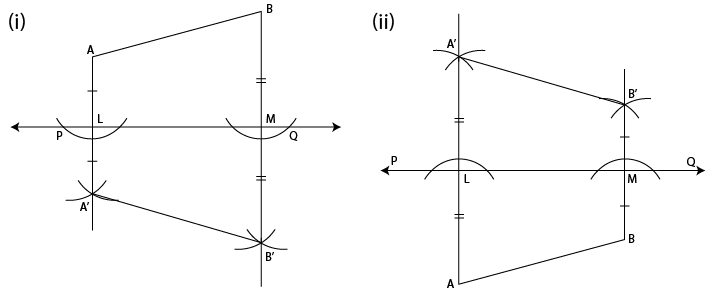Steps of Construction

(i) From the points A and B construct perpendiculars on PQ which intersects PQ at the points L and M.

(ii) Produce AL to the point A’ such that AL = LA’ and produce BM to B’ such that BM = MB’ A’B’ is the image of the line segment AB in PQ.

3. Complete the following table: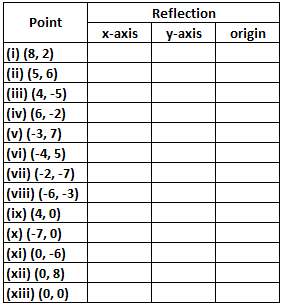Solution: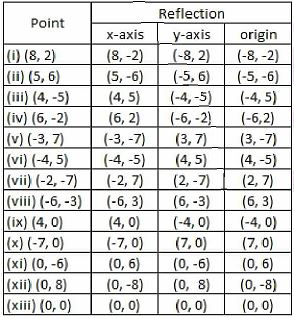4. A point P (7, 3) is reflected in x-axis to point P’. The point P’ is further reflected in y-axis to point P”. Find:

(i) the co-ordinates of P’

(ii) the co-ordinates of P”

(iii) the image of P (7, 3) in origin.

Solution:

(i) The image of point P (7, 3) when reflected in x-axis is P’ whose co-ordinates will be (7, -3).

(ii) The image of point P’ (7, -3) when reflected in y-axis P” whose co-ordinates will be (-7, -3).

(iii) The image of point P (7, 3) in the origin is P” whose co-ordinates are (-7, -3).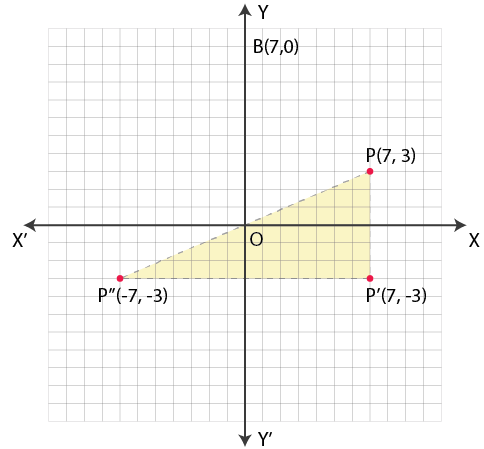5. A point A (- 5, 4) is reflected in y-axis to point B. The point B is further reflected in origin to point C. Find:

(i) the co-ordinates of B

(ii) the co-ordinates of C

(iii) the image of A (- 5, 4) in x-axis.

Solution:

(i) The image of point A (-5, 4) when reflected in y-axis is B whose co-ordinates will be (5, 4).

(ii) The image of point B (5, 4) when reflected in origin is C whose co-ordinates will be (-5, -4).

(iii) The image of point A (-5, 4) in x-axis is C whose co-ordinates are (-5, -4).6. The point P (3, – 8) is reflected in origin to point Q. The Point Q is further reflected in x-axis to point R. Find:

(i) the co-ordinates of Q

(ii) the co-ordinates of R

(iii) the image of P (3, – 8) in y-axis.

Solution:

(i) The image of point P (3, -8) when reflected in origin is Q whose co-ordinates will be (-3, 8).

(ii) The image of point Q (-3, 8) when reflected in x-axis is R whose co-ordinates will be (-3, -8).

(iii) The image of point P (3, -8) in y-axis is R whose co-ordinates are (-3, -8).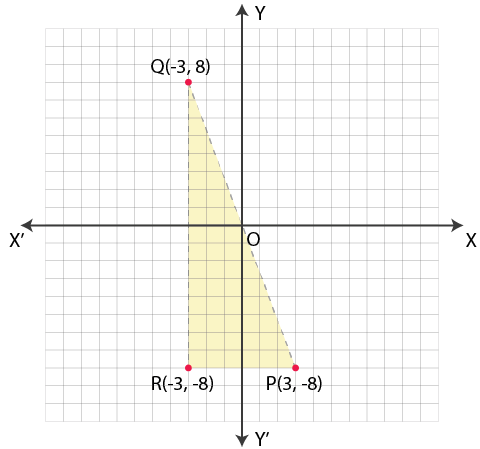7. Each of the points A (3, 0), B (7, 0), C (- 8, 0), D (- 7, 0) and E (0, 0) is reflected in x-axis to points A’, B’, C’, D’ and E’ respectively. Write the co-ordinates of each of the image points A’, B’, C’, D’ and E’.

Solution:

The points given are:

A (3, 0), B (7, 0), C (- 8, 0), D (- 7, 0) and E (0, 0)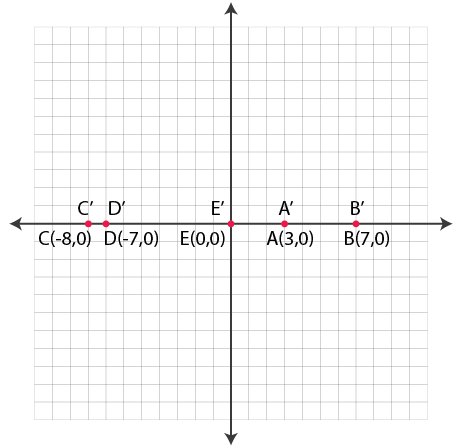The images will be reflected in x-axis

A’ (3, 0), B’ (7, 0), C’ (-8, 0), D’ (-7, 0) and E’ (0, 0) as the points lie on x-axis.

8. Each of the points A (0, 4), B (0, 10), C (0, – 4), D (0, – 6) and E (0, 0) is reflected in y-axis to points A’, B’, C’, D’ and E’ respectively. Write the co-ordinates of each of the image points A’, B’, C’, D’ and E’.

Solution:

The points given are

A (0, 4), B (0, 10), C (0, – 4), D (0, – 6) and E (0, 0) which are reflected on y-axis

The co-ordinates of their images will be

A’ (0, 4), B’ (0, 10), C’ (0, -4), D’ (0, -6) and E’ (0, 0) as they lie on y-axis.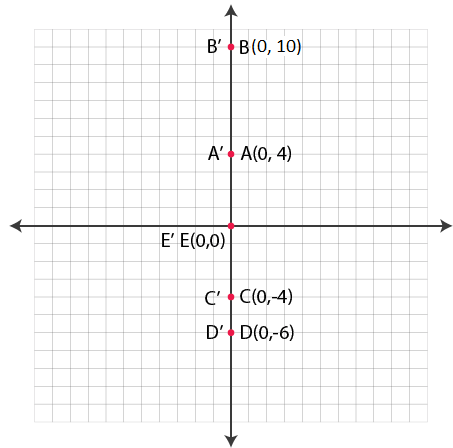9. Each of the points A (0, 7), B (8, 0), C (0, -5), D (- 7, 0) and E (0, 0) are reflected in origin to points A’, B’, C’, D’ and E’ respectively. Write the co-ordinates of each of the image points A’, B’, C’, D’ and E’.

Solution:

It is given that

A (0, 7), B (8, 0), C (0, -5), D (- 7, 0) and E (0, 0) are reflected in origin

The co-ordinates of their images will be

A’ (0, -7), B’ (-8, 0), C’ (0, 5), D’ (7, 0) and E’ (0, 0)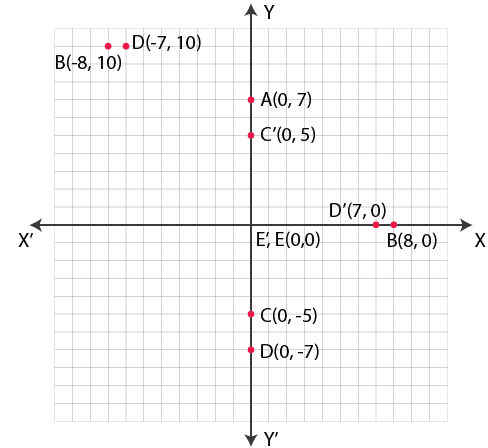10. Mark points A (4, 5) and B (- 5, 4) on a graph paper. Find A’, the image of A in x-axis and B’, the image of B in x-axis. Mark A’ and B’ also on the same graph paper. Join AB and A’ B’ and find if AB = A’ B’?

Solution:

It is given that

A (4, 5) and B (- 5, 4) are marked on the graph.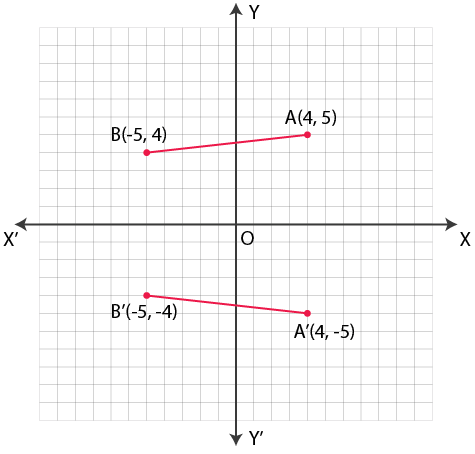The image of A in x-axis A (4, -5) and the image of B in x-axis is B’ (-5, -4) which are plotted on the same graph.

AB and A’B’ are joined.

Here AB = A’B’.

11. Mark points A (6, 4) and B (4, – 6) on a graph paper. Find A’, the image of A in y-axis and B’, the image of B in y-axis. Mark A’ and B’ also on the same graph paper.

Solution:

The points given are

A (6, 4) and B (4, – 6)

The images of A and B in y-axis are

A’ (-6, 4) and B’ (-4, -6) as shown in the graph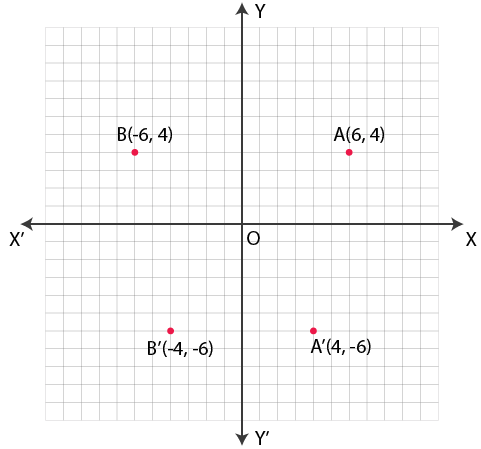12. Mark points A (- 6, 5) and B (- 4, – 6) on a graph paper. Find A’, the image of A in origin and B’, the image of B in origin. Mark A’ and B’ also on the same graph paper. Join AB and A’ B’ and find if AB = A’ B’ ?

Solution:

The points given are

A (- 6, 5) and B (- 4, – 6)

The images of A and B in the origin are A’ and B’

The co-ordinates are A’ (6, -5) and B’ (4, 6) which are plotted in the graph.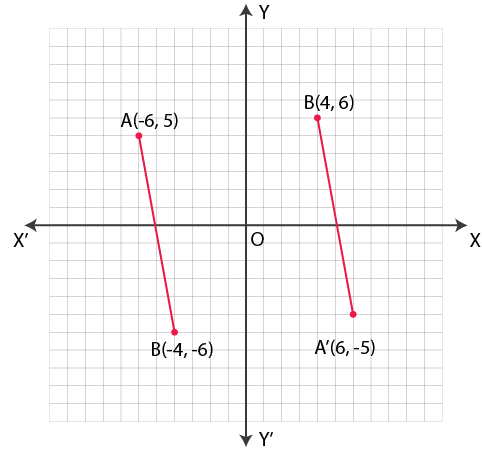Join AB and A’B’.

Here AB = A’B’.

#### Exercise 17C page: 201

1. How many lines of symmetry does a rhombus have?

Solution:

A rhombus has two lines of symmetry.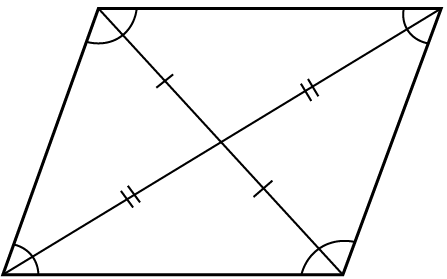2. What is the order of rotational symmetry of a rhombus?

Solution:

The number of times a shape appears exactly the same during full rotation is called the order of rotational symmetry.

The order of rotational symmetry of a rhombus is 2.

3. Show that each of the following figures has two lines of symmetry and a rotational symmetry of order 2.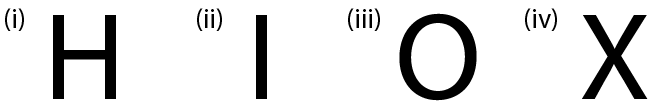Solution: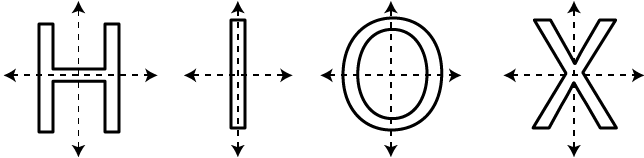4. Name a figure that has a line of symmetry but does not have any rotational symmetry.

Solution:

A kite shaped figure has one line of symmetry and no rotational symmetry.5. In each of the following figures, draw all possible lines of symmetry and also write the order of rotational symmetry: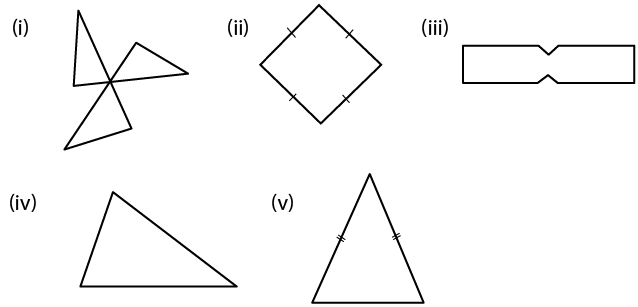Solution:

(i) No line of symmetry

The order of rotational symmetry is 3.

(ii) 2 line of symmetry

The order of rotational symmetry is 2.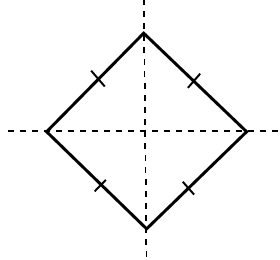(iii) 2 line of symmetry

The order of rotational symmetry is 2.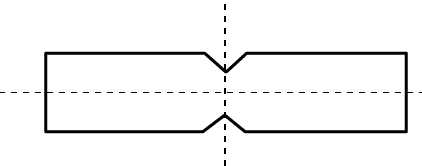(iv) No line of symmetry

The order of rotational symmetry is 0.

(v) 1 line of symmetry

The order of rotational symmetry is 0.